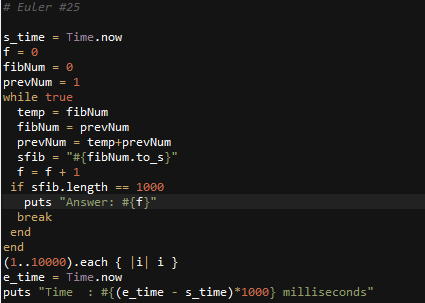# Ruby: Solution to Project Euler Problem # 25

Recently, I’ve been typing more Ruby then usual. In Ruby you can have a fairly big number.
http://projecteuler.net/problem=25

1000-digit Fibonacci number

The Fibonacci sequence is defined by the recurrence relation:

Fn = Fn1 + Fn2, where F1 = 1 and F2 = 1.

The 12th term, F12, is the first term to contain three digits.

What is the first term in the Fibonacci sequence to contain 1000 digits?